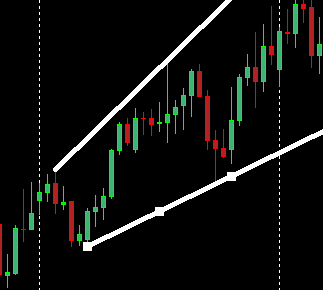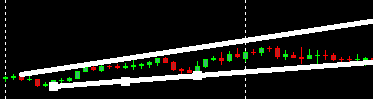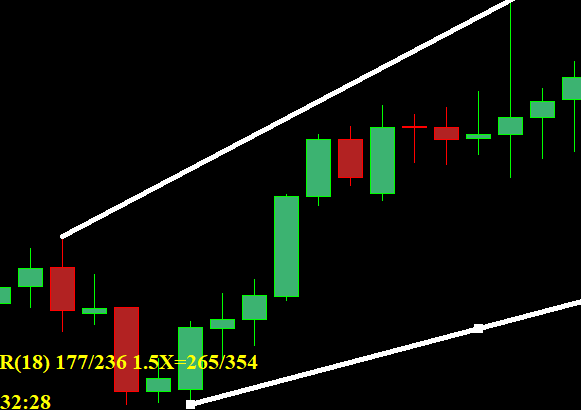Cant find and use trendline angle - page 3315

zemo:
My take is measuring the difference between the upperBB and lowerBB and display it as an indicator then applying the angle measurement on this indicator.198

Tom Sasson:

I am sorry to bring this post up, I just couldn't find the answer here and it bothered me to see answers like "it can't be done" etc.

So, it CAN be done, using my example below.

First I will explain what needs to be done, since we all agree that the angle cannot be determined if your coordinates are (price,time), so :

1. First challenge is that you need to change them to the chart's (x,y), so we'll get what we see visually.

2. Second challenge is that (x,y) are also not on the same scale, so you need to multiply your resulted slope by the width/height ratio.

So the code should be something like:

i have tested it ! it runs very well in the real-time chart,but failed in the stratege tester.it resulted in a critical error as the file below, i don't know why!

Files:
meafu2.jpg 11 kb18880

Tom Sasson: I just couldn't find the answer here and it bothered me to see answers like "it can't be done" etc. you need to multiply your resulted slope by the width/height ratio.

These three images with two lines are the same chart. Are the angles the same? No, because of the chart scaling. Angles are meaningless.

Multiplying slope by the height and width scale means you get different answers for the same slope - meaningless.